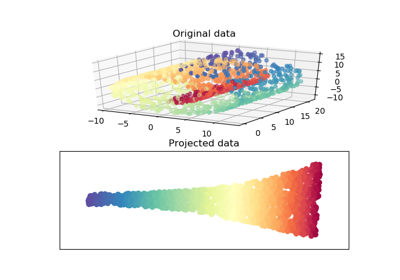# sklearn.manifold.locally_linear_embedding¶

sklearn.manifold.locally_linear_embedding(X, n_neighbors, n_components, reg=0.001, eigen_solver='auto', tol=1e-06, max_iter=100, method='standard', hessian_tol=0.0001, modified_tol=1e-12, random_state=None, n_jobs=None)[source]

Perform a Locally Linear Embedding analysis on the data.

Read more in the User Guide.

Parameters: X : {array-like, NearestNeighbors} Sample data, shape = (n_samples, n_features), in the form of a numpy array or a NearestNeighbors object. n_neighbors : integer number of neighbors to consider for each point. n_components : integer number of coordinates for the manifold. reg : float regularization constant, multiplies the trace of the local covariance matrix of the distances. eigen_solver : string, {‘auto’, ‘arpack’, ‘dense’} auto : algorithm will attempt to choose the best method for input data arpack : use arnoldi iteration in shift-invert mode. For this method, M may be a dense matrix, sparse matrix, or general linear operator. Warning: ARPACK can be unstable for some problems. It is best to try several random seeds in order to check results. dense : use standard dense matrix operations for the eigenvalue decomposition. For this method, M must be an array or matrix type. This method should be avoided for large problems. tol : float, optional Tolerance for ‘arpack’ method Not used if eigen_solver==’dense’. max_iter : integer maximum number of iterations for the arpack solver. method : {‘standard’, ‘hessian’, ‘modified’, ‘ltsa’} standard : use the standard locally linear embedding algorithm. see reference  hessian : use the Hessian eigenmap method. This method requires n_neighbors > n_components * (1 + (n_components + 1) / 2. see reference  modified : use the modified locally linear embedding algorithm. see reference  ltsa : use local tangent space alignment algorithm see reference  hessian_tol : float, optional Tolerance for Hessian eigenmapping method. Only used if method == ‘hessian’ modified_tol : float, optional Tolerance for modified LLE method. Only used if method == ‘modified’ random_state : int, RandomState instance or None, optional (default=None) If int, random_state is the seed used by the random number generator; If RandomState instance, random_state is the random number generator; If None, the random number generator is the RandomState instance used by np.random. Used when solver == ‘arpack’. n_jobs : int or None, optional (default=None) The number of parallel jobs to run for neighbors search. None means 1 unless in a joblib.parallel_backend context. -1 means using all processors. See Glossary for more details. Y : array-like, shape [n_samples, n_components] Embedding vectors. squared_error : float Reconstruction error for the embedding vectors. Equivalent to norm(Y - W Y, 'fro')**2, where W are the reconstruction weights.

References

  (1, 2) Roweis, S. & Saul, L. Nonlinear dimensionality reduction by locally linear embedding. Science 290:2323 (2000).
  (1, 2) Donoho, D. & Grimes, C. Hessian eigenmaps: Locally linear embedding techniques for high-dimensional data. Proc Natl Acad Sci U S A. 100:5591 (2003).
  (1, 2) Zhang, Z. & Wang, J. MLLE: Modified Locally Linear Embedding Using Multiple Weights. http://citeseerx.ist.psu.edu/viewdoc/summary?doi=10.1.1.70.382
  (1, 2) Zhang, Z. & Zha, H. Principal manifolds and nonlinear dimensionality reduction via tangent space alignment. Journal of Shanghai Univ. 8:406 (2004)

## Examples using sklearn.manifold.locally_linear_embedding¶Swiss Roll reduction with LLE